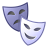# Help to find file index based on year, month and week in Python

258
0
09-11-2017 07:20 AMNew Contributor III

Hi,

I need to find the file index from a list of rasters that matches year, month and week. My code below work when I give the index manually.  But, I need to loop through many raster files.

My fmaskrasters list has 180 rasters and as follows:

fmaskrasters = [

Week_1_Sum1981_10_Crawford.tif

Week_1_Sum1982_10_Crawford.tif

.

Week_1_Sum2016_10_Crawford.tif

Week_2_Sum1981_10_Crawford.tif

.

Week_2_Sum2016_10_Crawford.tif

.

Week_5_Sum2016_10_Crawford.tif]

normal raster list has 5 rasters).

normal = [Crawford_Normal_Week_1_1981_2016_10.tif,

Crawford_Normal_Week_2_1981_2016_10.tif,

.

Crawford_Normal_Week_5_1981_2016_10.tif]

Can somebody help me to find the index from each raster list that matches, year, month and week so that I calculate the percent difference. My code below works fine but I need to pass the index that matches year, month and week criteria to find the index of raster from "fmaskrasters" and "normal".

Thanks in advance,

``months = weeks = years = arcpy.env.workspace = r'H:\PRISM_800m_weekly_sum\Crawford\All' out = r'H:\PRISM_800m_weekly_sum\Crawford\All\output'for year in years: for month in months: for week in weeks: arcpy.env.workspace = r'H:\PRISM_800m_weekly_sum\Crawford\All' fmaskrasters = arcpy.ListRasters() fmaskrasters.sort() #"Week_" + str(week) + "_Sum" + str(year) + "_" + str(month) + "_Crawford.tif" #for file in fmaskrasters: # if fnmatch.fnmatch(file, 'Week_' + str(week) + '_Sum' + str(year) + '_' + str(month) + '_Crawford.tif'): # print (file) fmaskrasters1=fmaskrasters weekras1 = arcpy.Raster(fmaskrasters1)  arcpy.env.workspace = r'H:\PRISM_800m_weekly_sum\Crawford\All\output'  normal = arcpy.ListRasters() normal.sort() #normalras = arcpy.Raster(normal["Crawford_Normal_Week_" + str(week) + "_1981_2016_" + str(month) + ".tif"]) normalras1=normal outcalc1 = Minus(normalras1, weekras1)  Final = (outcalc1/normalras1)*100 finras1 = out + "\\" + "Crawford_Normal_Week_" + str(week) + "_Difference" + "_" + str(year) + "_" + str(month) + ".tif"arcpy.CopyRaster_management(Final,finras1 + "","","","","","")‍‍‍‍‍‍‍‍‍‍‍‍‍‍‍‍‍‍‍‍‍‍‍‍‍‍‍‍‍‍``
Tags (3)
0 Replies